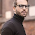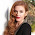# Longest Common Sub-sequence (LCS) Using C++

The longest common sub-sequence (LCS) problem is the problem of finding the longest sub-sequence common to all sequences in a set of sequences (often just two sequences). It differs from problems of finding common sub-strings: unlike sub-strings, sub-sequences are not required to occupy consecutive positions within the original sequences. The longest common sub-sequence problem is a classic computer science problem, the basis of data comparison programs such as the diff utility, and has applications in bioinformatics. It is also widely used by revision control systems such as Git for reconciling multiple changes made to a revision-controlled collection of files.

// C++ Program to implement Longest Common Sub-sequence(LCS).

```#include<iostream>

using namespace std;

int strlen(char []);
int max(int a, int b);

int lcs( char *X, char *Y, int m, int n )
{
if (m == 0 || n == 0)
return 0;
if ((X[m] == Y[n])&&(m>0&&n>0))
return 1 + lcs(X, Y, m-1, n-1);
else
return max(lcs(X, Y, m, n-1), lcs(X, Y, m-1, n));
}

int max(int a, int b)
{
if(a>b)
{
return a;
}
else
{
return b;
}
}

int strlen(char s[])
{
int p;
for(p=1; s[p]!='\0'; p++);
return p;
}

int main()
{
char X[] = "abcbdab";
char Y[] = "bdcaba";

int m = strlen(X);
cout<<endl<<"Length of X[] is = "<<m<<endl;
int n = strlen(Y);
cout<<endl<<"Length of Y[] is = "<<n<<endl;

cout<<endl<<"Length of LCS is = "<<lcs( X, Y, m, n )<<endl;
return 0;
}
```

// Output of the above program.

1.Can you print the subsequence ?

2.Can u provide me the code for printing LCS of sequence of integer number

3.This is so needful article.thanks for sharing with us.

4.It was an amazing article.Thanks a lot for sharing this

5.Awesome way to present photography. Love it most. Keep it up. Thanks